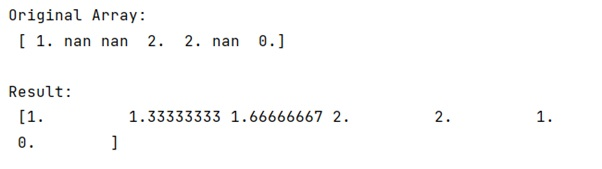# Interpolate NaN values in a numpy array

Learn, how to interpolate NaN values in a numpy array in Python?
Submitted by Pranit Sharma, on January 21, 2023

NumPy is an abbreviated form of Numerical Python. It is used for different types of scientific operations in python. Numpy is a vast library in python which is used for almost every kind of scientific or mathematical operation. It is itself an array which is a collection of various methods and functions for processing the arrays.

## NumPy Array - Interpolating NaN Values

Suppose that we are given a numpy array that contains some numerical values and some nan values and we need to find a way to replace the nan values of this array with the linearly interpolated values of this array.

While creating a DataFrame or importing a CSV file, there could be some NaN values in the cells. NaN values mean "Not a Number" which generally means that there are some missing values in the cell.

For this purpose, we will first find the index of all the non-nan values and then extract all the non-zero values from them. We will then use numpy.interp() method. This method returns the one-dimensional piecewise linear interpolant to a function with given discrete data points (xp, fp), evaluated at x.

Let us understand with the help of an example,

## Python code to interpolate NaN values in a numpy array

```import numpy as np
nan = np.nan

# Creating a numpy array
arr = np.array([1, nan, nan, 2, 2, nan, 0])

# Display original array
print("Original Array:\n",arr,"\n")

# Making sequences for interp
ok = ~np.isnan(arr)
xp = ok.ravel().nonzero()
fp = arr[~np.isnan(arr)]
x  = np.isnan(arr).ravel().nonzero()

# Replacing nan values
arr[np.isnan(arr)] = np.interp(x, xp, fp)

# Display result
print ("Result:\n",arr)
```

Output:What's New (MCQs)

Top Interview Coding Problems/Challenges!

IncludeHelp's Blogs

Languages: » C » C++ » C++ STL » Java » Data Structure » C#.Net » Android » Kotlin » SQL
Web Technologies: » PHP » Python » JavaScript » CSS » Ajax » Node.js » Web programming/HTML
Solved programs: » C » C++ » DS » Java » C#
Aptitude que. & ans.: » C » C++ » Java » DBMS
Interview que. & ans.: » C » Embedded C » Java » SEO » HR
CS Subjects: » CS Basics » O.S. » Networks » DBMS » Embedded Systems » Cloud Computing
» Machine learning » CS Organizations » Linux » DOS
More: » Articles » Puzzles » News/Updates